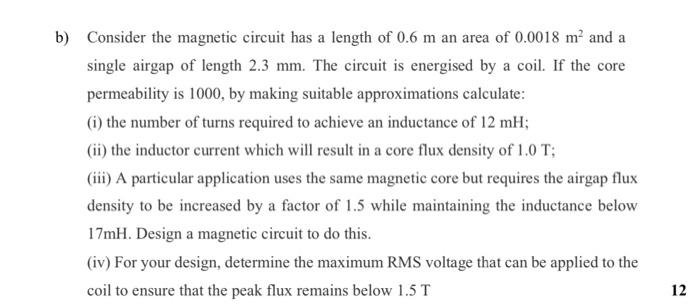# Question Solved1 Answerb) (i) N = 110 turns; (ii) IL = 16.5 AThese are the finalanswer Of this Question so please make sure everything 100% make sure answers are same i want to learn the exact method so please make sure everything 100% b) Consider the magnetic circuit has a length of 0.6 m an area of 0.0018 m' and a single airgap of length 2.3 mm. The circuit is energised by a coil. If the core permeability is 1000, by making suitable approximations calculate: (i) the number of turns required to achieve an inductance of 12 mH; (ii) the inductor current which will result in a core flux density of 1.0 T; (iii) A particular application uses the same magnetic core but requires the airgap flux density to be increased by a factor of 1.5 while maintaining the inductance below 17mH. Design a magnetic circuit to do this. (iv) For your design, determine the maximum RMS voltage that can be applied to the coil to ensure that the peak flux remains below 1.5 T 12DRIOEO The Asker · Electrical Engineering
b) (i) N = 110 turns; (ii) IL = 16.5 A
These are the final
answer Of this Question so please make sure everything 100% make sure answers are same i want to learn the exact method so please make sure everything 100%Transcribed Image Text: b) Consider the magnetic circuit has a length of 0.6 m an area of 0.0018 m' and a single airgap of length 2.3 mm. The circuit is energised by a coil. If the core permeability is 1000, by making suitable approximations calculate: (i) the number of turns required to achieve an inductance of 12 mH; (ii) the inductor current which will result in a core flux density of 1.0 T; (iii) A particular application uses the same magnetic core but requires the airgap flux density to be increased by a factor of 1.5 while maintaining the inductance below 17mH. Design a magnetic circuit to do this. (iv) For your design, determine the maximum RMS voltage that can be applied to the coil to ensure that the peak flux remains below 1.5 T 12
More
Transcribed Image Text: b) Consider the magnetic circuit has a length of 0.6 m an area of 0.0018 m' and a single airgap of length 2.3 mm. The circuit is energised by a coil. If the core permeability is 1000, by making suitable approximations calculate: (i) the number of turns required to achieve an inductance of 12 mH; (ii) the inductor current which will result in a core flux density of 1.0 T; (iii) A particular application uses the same magnetic core but requires the airgap flux density to be increased by a factor of 1.5 while maintaining the inductance below 17mH. Design a magnetic circuit to do this. (iv) For your design, determine the maximum RMS voltage that can be applied to the coil to ensure that the peak flux remains below 1.5 T 12